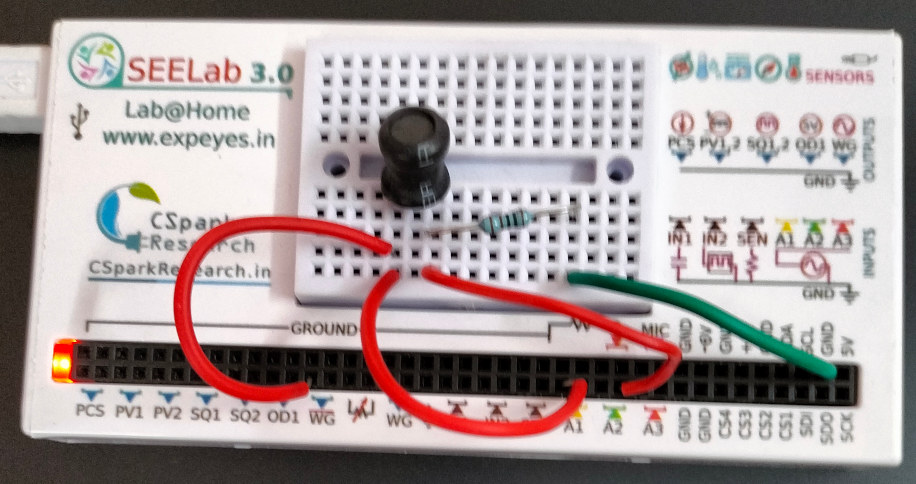# ExpEYES

## Inductor in AC Circuits

The objective of this experiment is to explore the phase relatioships of voltage and current across an inductor in an AC circuit. Inductive reactance and inductance are calculated from the measured values of current and voltage. The 1K resistor and A2 constitutes our ammeter.

• Connect a 10 mH inductor and a 1000 Ohm resistor in series from WG to ground.
• Connect A1 to WG and A2 to the junction.
• Set the frequency to 4000 Hz
• Slide on the display to select an area, to get the readings.The results show that

• Current through the Inductor = 3.145 mA
• Voltage across the inductor = 0.808 volts
• Voltage is leading by 85 degrees. It should be 90 degrees but the resistance of the coil (20 Ohms) introduces this difference.

Inductive reactance, $X_L=\frac{V_L}{I_L}= 2\pi fL$

implies $L=\frac{V_L}{2\pi f I_L}$ = 10.2 mH

The experimental results tally with the theory.

Repeat the process for 5000 Hz and 3000 Hz. Using an inductor having very low ohmic resistance would give better results.